##### Electronics For Dummies, 3rd EditionWhen you have a good handle on Ohm's Law, you'll be ready to put it into practice. Ohm's Law is like a master key, unlocking the secrets to electronic circuits.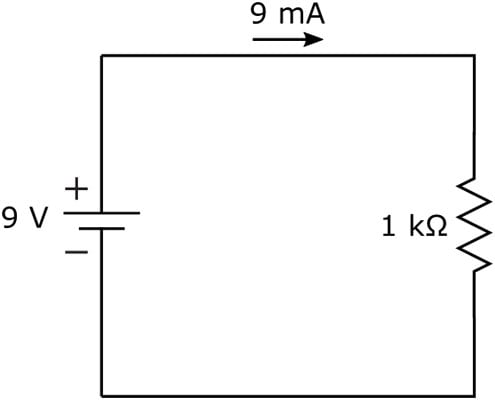In the simple circuit shown here, a 9-volt battery is applied across a 1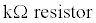You calculate the current through the resistor as follows: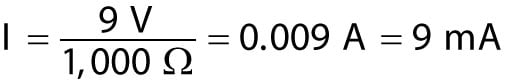If you add a 220 ohm resistor in series, as shown here, you're restricting the current even more.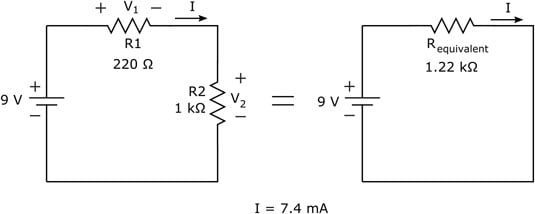To calculate the current through this circuit, determine the equivalent resistance and apply Ohm's Law.

To calculate the current flowing through the circuit, you need to determine the total resistance that the 9-volt battery is facing in the circuit. Because the resistors are in series, the resistances add up, for a total equivalent resistance of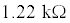You use this equivalent resistance to calculate the new current, as follows: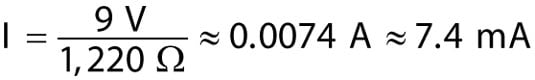By adding the extra resistor, you've reduced the current in your circuit from 9 mA to 7.4 mA.

That double squiggle symbol in the equation just given means "is approximately equal to," and it was used because the current was rounded to the nearest tenth of a milliamp. It's usually okay to round off the tinier parts of values in electronics — unless you're working on the electronics that control a subatomic particle smasher or other high-precision industrial device.